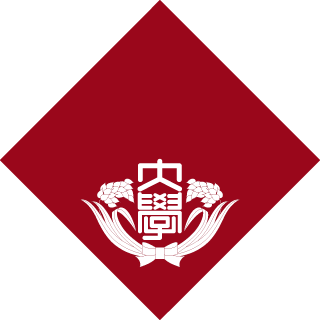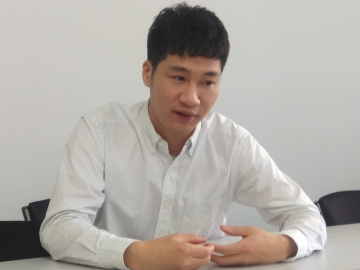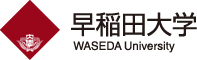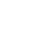#Waseda Institute for Advanced Study (WIAS)早稲田大学 高等研究所

ニュース

### Symmetry methods for discrete equations　PENG Linyu 講師### Longstanding interest

In 2004, I went to Beijing to do my undergraduate, and stayed there for six years before moving to the U.K. In the beginning I was in the department of computer science, but it didn’t really suit me. I transferred to mathematics after one and a half years’ study in electronic engineering.
At one point I started to learn differential geometry and there is a research field called geometric mechanics, in which mechanics is understood in a geometrical manner. I was interested in variational structures, especially Hamiltonian and Lagrangian mechanics. These preparations helped me to secure an opportunity to study for my PhD in the U.K. From October 2010 to July 2013, I worked on geometric and algebraic structures of differential and difference equations under the supervision of Professor Peter Hydon, who is currently at the University of Kent.

### Symmetry and conservation laws

One of my current research interests is related to Lie symmetries, for instance symmetries of continuous and discrete equations, variational problems and Noether conservation laws, moving frames, integrable systems, etc. Symmetry exists almost everywhere in our daily life. It can be precisely defined mathematically as an invariance property of a certain object. In particular, it is the key to solving differential equations, in which the set of solutions is invariant with respect to some group of symmetries.
My focus is in particular on discrete equations. There are many differential equations that we cannot solve analytically when numerical methods provide us alternative solutions. To propose efficient numerical methods, it is then essential to study the geometric and algebraic structures of the proposed numerical schemes, e.g. the preservation of symmetries or conservation laws. Discrete equations, however, can also rise up as dynamical models describing mechanical or physical systems.
A given differential equation induces a constrained variational co-chain complex of differential forms on jet bundles. Cohomology of such complexes can be determined using spectral sequences, for example; the set of nontrivial conservation laws corresponds to one of the cohomology groups. We reformulated these ideas using exact couples, enabling us to determine their discrete counterparts. In particular, Noether’s first theorem for both continuous and discrete equations, connecting symmetries for variational problems and conservation laws of the resulting Euler–Lagrange systems, can be interpreted in a coordinate-free manner. The complex is also closely related to inverse problems as well as symplectic and multisymplectic structures of variational equations.
Lie symmetry analysis for semi-discrete equations still needs further improvements, nevertheless. The situation becomes more complicated somehow due to the non-commutativity of differential and difference operators. Everything becomes trivial when regular symmetries are considered; it is important, however, to realize the importance of irregular symmetries and to achieve the most general symmetry theory. Next, it will be a good time to extend Noether’s theorem.

### The merging of pure and applied mathematics

This field lies between pure and applied mathematics. For instance, symmetries and conservation laws are closely related to algebraic geometry and moving frames, while they are widely applicable to other fields of mathematics, physics and engineering, e.g. field theory, fluid mechanics and integrable systems.
Since moving to Waseda, my knowledge has been expanded, in particular in fluid mechanics. Symmetry theory has the potential to provide further insights to analyze complex fluid systems we encounter. For instance, the formal Lagrangian approach may be able to help us understand inverse problems of the Navier—Stokes equation.

As a junior mathematician, it is usually important (or perhaps necessary) to move around the world, to widen your horizons. After I finished my PhD at England, I moved to Tokyo, starting with a JST postdoctoral position. The current position at WIAS offered a chance to continue my research in Japan and it’s turned out to be an excellent experience.

## Related Articles 関連記事

New
,
, ,
,
,
Page Top

#### www.waseda.jp/inst/wiasこのままご覧いただく方は、「このまま進む」ボタンをクリックし、次ページに進んでください。•Windowsバージョン38 以上
Macintoshバージョン38 以上
Webサイト

•Fire Fox
Windowsバージョン33 以上
Macintoshバージョン33 以上
Webサイト

•Safari
Windowsバージョン38 以上
Macintoshバージョン38 以上
Webサイト

•Internet Explorler
Windowsバージョン10 以上
Webサイト